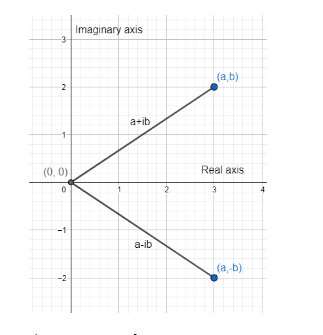QuestionAnswers

# Write the complex conjugate of 3 + 4i.Verified
128.7k+ views
Hint: To solve this question, first of all, we will define what are complex numbers and what are its conjugates with their implications on the graph of the complex number and its conjugate in the complex plane. Then, with the concepts we have learned, we will find the conjugate of 3 + 4i.

A complex number is just addition of two parts, one real and imaginary, in the form a + ib. where, ${{i}^{2}}$ = ─1. Since, this value of i doesn’t exist for any real number, mathematicians have defined complex numbers. The complex number a + ib has an imaginary part as well as a real part. a in the complex number a + ib is real whereas b in the complex number is an imaginary part. Either of a or b can be 0.